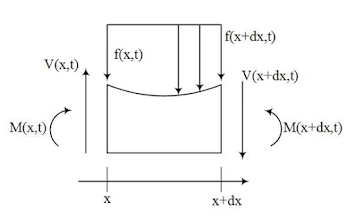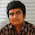## Welcome to the World of Modelling and Simulation

### What is Modelling?

This blog is all about system dynamics modelling and simulation applied in the engineering field, especially mechanical, electrical, and ...### Euler-Bernoulli Beam Equation Solution by a Finite Difference Approach

In this article, at first, we discuss the concept of the very famous, widely used Euler-Bernoulli beam theory. We apply the theoretical development to a wind turbine blade since the blade may be represented as a cantilever beam, where one end is fixed to the hub, and the other end is free. Next, we develop a finite difference scheme to solve the Euler-Bernoulli beam equation. Figure 1 shows a simple sketch for a wind turbine blade. This blade may be represented by a cantilever beam for its dynamics analysis. The blade is subjected to the distributed load 𝑓(𝑥, 𝑡), which may vary in time, and this is expressed as a force per unit length of the blade. The deflection of the blade in the vertical direction is expressed by 𝑤(𝑥, 𝑡), which depends on space and time.Figure 1: Uniform Bernoulli-Euler beam with distributed load.

The following assumptions are made for the mathematical model of the wind turbine blade .
• The blade deflects only in the vertical direction where the deflection is small.
• The slope is also small.
• Any planar cross-section of the blade remains planar when it is deflected. Newton’s law in the vertical direction for a small segment of the blade gives,Figure 2: Small segment of the blade.

Here, 𝜌 is the density of the material, 𝐴 is the cross-section of the blade, and 𝑉(𝑥,𝑡) is the shear force acting on the blade.

Now, expanding the terms in the Taylor series about x,
Substituting these into equation (1) and neglecting higher order terms gives,

Computing the moments about the center of the right end of the blade segment in figure 2 gives,

Where, the moment due to the loading is approximated as the average load with an average moment arm of dx/2.
Using again Taylor series expansion for M and f,

Substituting these into equation (3) and taking limit as 𝑑x →0 gives,

Substituting 𝑉(𝑥,𝑡) into equation (2),

From the elementary theory of the bending of a beam, the relationship between bending moment and deflection may be expressed as,

By substituting 𝑀(𝑥,𝑡) into equation (4),

Equation (5) represents the motion of the blade which is mostly known as Euler-Bernoulli beam equation.

Here, C² = EI / ⍴A.
E is the Young’s modulus, and I is the area moment of inertia.
Since, equation (6) involves fourth order in space and second order in time derivatives, solving equation (6) requires six conditions, in which two are the initial and four are the boundary conditions.

Where, f(x) is any displacement function. For our wind turbine blade, we will see the deflection of the blade due to the various defined initial functions.

Force-free Boundary Conditions:
The force-free boundary conditions are such that where there is no shear force and no moment exist at x=0 and x=L, where L is the length of the blade .

So, for the zero moment consideration, we get:

And, for the zero shear condition, we have:

## Finite Difference Method

To solve the problem numerically, we chose the finite difference approach. Figure 3 describes the computational domain with the implied initial and boundary conditions.Figure 3: Uniform finite difference grids for CTCS method.

Applying centered finite divided difference formulas for both in space and time or centered time centered space (CTCS) converts equation (6) as [2-3],

Now, assuming,

So, we have,

Equation (7) is the final difference equation for our system differential equations. This scheme is conditionally stable as it depends on the term (2−6𝑘). The condition for stability is,

2−6𝑘≥0

We also have three points outside our computational domain in equation (7) which are 𝑓𝑖, 𝑗−1, 𝑓𝑖−1, 𝑗, and 𝑓𝑖−2, 𝑗. The boundary conditions for this problem will help to relate these points with some other points in the domain.

In figure 3, the rows represent time and columns represent space. In the first row when j=1 (i.e. time is zero), the displacement function 𝑓(𝑥) is used to calculate all the points in the computational domain. Equation (7) finds the deflection from the second row up to the defined computational domain.

The first derivative for the initial condition when t=0 may be approximated as,

The second derivative for the boundary condition at x=0 may be approximated as,

For our problem, second derivative 𝑤_𝑥𝑥 (𝑖,𝑗) (0, 𝑡) at x=0 is zero.
Substituting 𝑓𝑖−1, 𝑗 into equation (7) gives,

Now, the third derivative for the boundary condition at x=0 may be approximated as,

Again, for our problem the third derivative 𝑤_𝑥x𝑥 (𝑖, 𝑗) (0, 𝑡) is zero.
Substituting 𝑓𝑖−2, 𝑗 into equation (8) gives,

Equation (9) is used to calculate first point of second row in the computational domain on the left end boundary (x=0). In the remaining rows, first point is calculated as,

For calculating the second point of the second row following relation is used which is obtained by replacing (i) with (i+1) in equation (7) after some manipulations.

In the remaining rows, second point is calculated as,
After these steps, equation (7) is used to find out rest of the points of the second row in the domain until the point just from the right end boundary (x=L) is reached. And, for this point and the point on the right end boundary, other two boundary conditions on space are implemented similar to the left end boundary (x=0) conditions to get the points beyond the computational domain.

The second derivative for the boundary condition at x=L may be approximated as,

Second derivative 𝑤𝑤_𝑥𝑥 (𝑖+6, 𝑗) (𝐿, 𝑡) at x=L is zero in the computation domain in figure 3.
The third derivative for the boundary condition at x=L may be approximated as,

Also, for our problem the third derivative 𝑤_𝑥x𝑥 (𝑖+6, 𝑗) (𝐿, 𝑡) is zero.
At point 𝑖+5, equation (7) may be written as,

Substituting 𝑓𝑖+7, 𝑗 into equation above gives,

Equation (13) is used to calculate last point of second row in the computational domain just from the right end boundary (x=L). In the remaining rows, this point is calculated as,

At point 𝑖+6, equation (9) may be written as,

Substituting 𝑓𝑖+7, 𝑗 and 𝑓𝑖+8, 𝑗 into equation (15) gives,

Equation (16) is used to calculate the point of the second row on the right end boundary (x=L) in the computational domain. For the remaining points,

## Results and Discussions

We developed a MATLAB program for this numerical solution technique. The detailed code with explanations is given in the appendix A.
The initial deflection or displacement function for this problem is defined as a sinusoidal function, which is expressed as 𝑓(𝑥) = sin𝑥 + sin2𝜋𝑥, where 𝑡=0
And at this moment, the initial velocity function is zero. So, 𝑔(𝑥) = 0.

Now, the uniform grid difference or step size is taken as ℎ=0.05. We will explore the results for different values of k in equation (7) which plays a vital role in this particular dynamics. Basically, k is the function of the blade material property (modulus of elasticity, moment of inertia, density, and so on), geometry and step size of the considered finite difference scheme. In the following, the effects of changing this parameter on the blade deflection are enlightened.

When k = 0.001:

When k = 0.01:

When k = 0.05:
When k = 0.1:

By observing the above plots for increasing values of k shows that the blade deflection after some time from simulation inception is approaching zero; however, there is a large deflection at the initial period, which suggests that by further increasing the value of k makes the system unstable, which also satisfies our stability criterion defined in the derivation. And it is apparent that keeping lower magnitude of k gives the reasonable amount of defection from the initial period, which then propagates throughout the blade with increase in time.

## References

 Bill Goodwine, Engineering Differential Equations: Theory and Applications, DOI 10.1007/978-1-4419-7919-3.
 Numerical methods for engineers / Steven C. Chapra, Raymond P. Canale. — 6th ed., published by McGraw-Hill Companies, Inc., 2010.
 Hoffman, J., Numerical Methods for Engineers and Scientists, McGraw-Hill, New York, 1992.
 D. C. Karnopp, D. L. Margolis, and R. C. Rosenberg, System Dynamics: Modeling and Simulation of Mechatronic Systems, John Wiley and Sons Ltd. (Fourth Edition).

## APPENDIX A

function U=problemone(m,n)
h=0.1; % Uniform interval
k=0.1; % Constant influences system responses
U=zeros(m,n); % Solution Matrix initialization
% First row generation
for i=1:n
f=@(U) sin(pi*(h*i))+sin(2*pi*(h*i)); % Initial condition,deflection
U(1,i)=feval(f,(h*i));
end
% Second row generation
for j=2
g=@(U) 0; % Initial condition,velocity
% First point of second row calculation
for i=1
U(j,i)=(1-k)*U(j-1,i)+2*k*U(j-1,i+1)+h*feval(g,h*(j-1))-k*U(j-1,i+2);
end
% Second point of second row calculation
for i=2
U(j,i)=(1-(2.5*k))*U(j-1,i+1)+2*k*U(j-1,i+2)+h*feval(g,h*(j))-0.5*k*U(j-1,i+3)+k*U(j-1,i);
end
% Remaining points of second row calculation
for i=3:n-2
U(j,i)=(2-(6*k))*U(j-1,i)-k*U(j-1,i+2)+4*k*U(j-1,i+1)+4*k*U(j-1,i-1)-k*U(j-1,i-2);
end
% Last point calculation just from the right end boundary of second row
for i=n-1
U(j,i)=(1-(2.5*k))*U(j-1,n-1)+2*k*U(j-1,n-2)+h*feval(g,h*(j-1))-0.5*k*U(j-1,n-3)+k*U(j-1,n);
end
% Point on the right end boundary calculation of second row
for i=n
U(j,i)=(1-k)*U(j,n)+2*k*U(j,n-1)+h*feval(g,h*(j))-k*U(j,n-2);
end
end
% Remaining rows generation of computational domain
for j=3:m
% First point of remaining rows calculation
for i=1
U(j,i)=((2-(2*k))*U(j-1,i))+((4*k)*U(j-1,i+1))-((2*k)*U(j-1,i+2))-U(j-1,i);
end
% Second point of remaining rows calculation
for i=2
U(j,i)=((2-(5*k))*U(j-1,i+1))+((4*k)*U(j-1,i+2))-(k*U(j-1,i+3))+((2*k)*U(j-1,i))-U(j-1,i);
end
% Remaining points of remaining rows calculation
for i=3:n-2
U(j,i)=((2-(6*k))*U(j-1,i))-(k*U(j-1,i+2))+((4*k)*U(j-1,i+1))+((4*k)*U(j-1,i-1))-(k*U(j-1,i-2))-U(j-1,i);
end
% Last point calculation just from the right end boundary of remaining rows
for i=n-1
U(j,i)=((2-(5*k))*U(j-1,n-1))+((4*k)*U(j-1,n-2))-(k*U(j-1,n-3))+((2*k)*U(j-1,n))-U(j-1,i);
end
% Point on the right end boundary calculation of remaining rows
for i=n
U(j,i)=((2-(2*k))*U(j-1,n))+((4*k)*U(j-1,n-1))-((2*k)*U(j-1,n-2))-U(j-1,i);
end
end
% Surface plot
figure (1)
surf(U);
xlabel('x');ylabel('t');zlabel('w(x,t)');
title('Deflection of Blade with respect to Space and Time')
figure (2)
plot(U(:,40));
xlabel('x');ylabel('w(x,t)');
title('Deflection of Blade at the Space End')
figure (3)
plot(U(40,:));
xlabel('t');ylabel('w(x,t)');
title('Deflection of Blade at the Final Time')

1.Do you have that same code in python?

2.Hi PM, unfortunately, I do not have in python.

3.Hi, how do you get equation(11)? I've tried to replacing (i) with (i+1) in equation (7), but still couldn't get (11).

1.Hi, I skipped some steps and showed the relation in final form. You need to substitute the relevant points in terms of others to get rid of the unknowns.

4.Hi, can you elaborate how to get eqn.(11)? Thanks

1.Hi, I skipped some steps and showed the relation in final form. You need to substitute the relevant points in terms of others to get rid of the unknowns.

2.Hi, I got equation(11) correctly, thanks. However, I have another question now, since the blade is represented by a cantilever beam, why using force-free boundary conditions as the moment and shear force at x=0 are not zero.

3.The boundary condition depends on your choice, for example, how you would see the solution or what you need in order to represent a physical phenomenon. I simply considered the boundary condition because of its convenience.